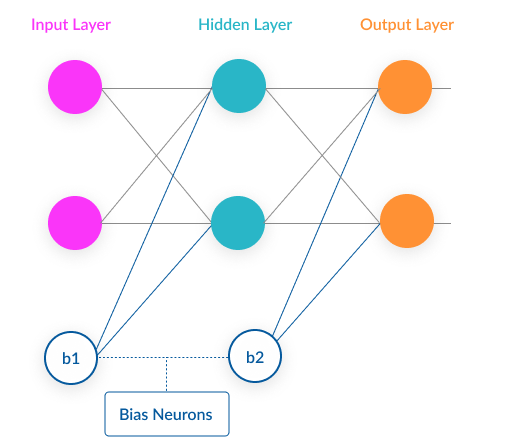/  Deep Learning Interview questions and answers   /  Explain Biases and Weights in Neural Network## Explain Biases and Weights in Neural Network

Ans: Bias is the Pre-assumption in a model. Bias is like the intercept added in a linear equation. It is an additional parameter which is used to adjust the output along with the weighted sum of the inputs to the neuron.

Weight is the steepness of the linear function. It is a strength of the connection. For increase in input how much influence it has in output. This is similar to slope in linear regression.